# The Quadratic Programming Solver

### Example 9.2 Portfolio Optimization

Consider a portfolio optimization example. The two competing goals of investment are (1) long-term growth of capital and (2) low risk. A good portfolio grows steadily without wild fluctuations in value. The Markowitz model is an optimization model for balancing the return and risk of a portfolio. The decision variables are the amounts invested in each asset. The objective is to minimize the variance of the portfolio’s total return, subject to the constraints that (1) the expected growth of the portfolio reaches at least some target level and (2) you do not invest more capital than you have.

Let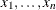be the amount invested in each asset,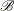be the amount of capital you have,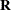be the random vector of asset returns over some period, andbe the expected value of. Let G be the minimum growth you hope to obtain, and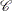be the covariance matrix of. The objective function is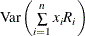, which can be equivalently denoted as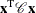.

Assume, for example, n = 4. Let= 10,000, G = 1,000,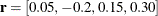, and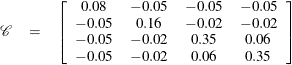The QP formulation can be written as: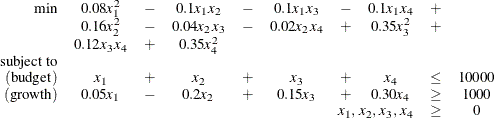Use the following SAS statements to solve the problem:

/* example 2: portfolio optimization */
proc optmodel;
/* let x1, x2, x3, x4 be the amount invested in each asset */
var x{1..4} >= 0;

num coeff{1..4, 1..4} = [0.08 -.05 -.05 -.05
-.05 0.16 -.02 -.02
-.05 -.02 0.35 0.06
-.05 -.02 0.06 0.35];
num r{1..4}=[0.05 -.20 0.15 0.30];

/* minimize the variance of the portfolio's total return */
minimize f = sum{i in 1..4, j in 1..4}coeff[i,j]*x[i]*x[j];

/* subject to the following constraints */
con BUDGET: sum{i in 1..4}x[i] <= 10000;
con GROWTH: sum{i in 1..4}r[i]*x[i] >= 1000;

solve with qp;

/* print the optimal solution */
print x;


The summaries and the optimal solution are shown in Output 9.2.1.

Output 9.2.1: Portfolio Optimization

The OPTMODEL Procedure

Problem Summary
Objective Sense Minimization
Objective Function f

Number of Variables 4
Bounded Above 0
Bounded Below 4
Bounded Below and Above 0
Free 0
Fixed 0

Number of Constraints 2
Linear LE (<=) 1
Linear EQ (=) 0
Linear GE (>=) 1
Linear Range 0

Constraint Coefficients 8

Performance Information
Execution Mode Single-Machine
Number of Threads 4

Solution Summary
Solver QP
Algorithm Interior Point
Objective Function f
Solution Status Optimal
Objective Value 2232313.4432

Primal Infeasibility 2.9198351E-9
Dual Infeasibility 1.9359348E-8
Bound Infeasibility 0
Duality Gap 1.3492254E-7
Complementarity 1.0600935E-7

Iterations 7
Presolve Time 0.00
Solution Time 0.02

 x
1 3452.9
2 0.0
3 1068.8
4 2223.5

Thus, the minimum variance portfolio that earns an expected return of at least 10% is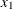= 3,452,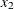= 0,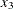= 1,068,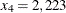. Asset 2 gets nothing because its expected return is20% and its covariance with the other assets is not sufficiently negative for it to bring any diversification benefits. What if you drop the nonnegativity assumption?

Financially, that means you are allowed to short-sell—that is, sell low-mean-return assets and use the proceeds to invest in high-mean-return assets. In other words, you put a negative portfolio weight in low-mean assets and more than 100% in high-mean assets.

To solve the portfolio optimization problem with the short-sale option, continue to submit the following SAS statements:

   /* example 2: portfolio optimization with short-sale option */
/* dropping nonnegativity assumption */
for {i in 1..4} x[i].lb=-x[i].ub;

solve with qp;

/* print the optimal solution */
print x;
quit;


You can see in the optimal solution displayed in Output 9.2.2 that the decision variable, denoting Asset 2, is equal to1,563.61, which means short sale of that asset.

Output 9.2.2: Portfolio Optimization with Short-Sale Option

The OPTMODEL Procedure

Solution Summary
Solver QP
Algorithm Interior Point
Objective Function f
Solution Status Optimal
Objective Value 1907122.2254

Primal Infeasibility 6.4988973E-8
Dual Infeasibility 7.3644141E-9
Bound Infeasibility 0
Duality Gap 2.0104247E-8
Complementarity 6.299141E-7

Iterations 5
Presolve Time 0.00
Solution Time 0.01

 x
1 1684.35
2 -1563.61
3 682.51
4 1668.95